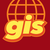# Return the largest value from a list

202
4
02-28-2020 01:35 PMNew Contributor III

I have a Python script that I am attempting to use to pass the largest value from a list and initialize a counter for sequential ID population.

It has been a long time since I've worked with updatecursors, so please pardon if my code is a bit rusty:

with arcpy.da.SearchCursor(fc, [fldID]) as rows:
myLst = []
for row in rows:
myLst.append(row)
intlist = [int(s) for s in myLst if s.isdigit()]
print intlist
highestval= max(intlist)
print int(highestval)

for myVal in myLst:
highestval = counter
counter += 1
with arcpy.da.UpdateCursor(fc, [fldID]) as rows:
for row in rows:
row = prefixName + counter

edit.stopOperation()
edit.stopEditing(True)
del cursor

The problem is, the ID field is a String and not an integer. an example is wFH0003. I am looking at just grabbing the digits from the string. my max(intlist) is returning an empty value. I want to use this as a script to populate ID's that are null or empty.

Any help would be appreciated.

1 Solution

Accepted SolutionsbyMVP Esteemed Contributor

``````# ---- now some sample data

a = ['a1', 'a100', None, 'a2']

# ---- now the case, especially if you have None

[i[len(i.rstrip('0123456789')):] for i in a if i]
['1', '100', '2']

# ---- if you need integers

[int(i[len(i.rstrip('0123456789')):]) for i in a if i]
[1, 100, 2]‍‍‍‍‍‍‍‍‍‍‍‍‍‍‍‍‍‍‍‍‍‍‍‍‍‍

# ---- you can obviously lstrip as well‍‍‍‍‍‍‍‍‍‍‍‍‍‍‍‍‍‍‍‍‍‍‍‍‍‍‍‍‍‍‍‍``````

And you just take the "max" of the returned list

4 RepliesbyMVP Esteemed Contributor

``````# ---- now some sample data

a = ['a1', 'a100', None, 'a2']

# ---- now the case, especially if you have None

[i[len(i.rstrip('0123456789')):] for i in a if i]
['1', '100', '2']

# ---- if you need integers

[int(i[len(i.rstrip('0123456789')):]) for i in a if i]
[1, 100, 2]‍‍‍‍‍‍‍‍‍‍‍‍‍‍‍‍‍‍‍‍‍‍‍‍‍‍

# ---- you can obviously lstrip as well‍‍‍‍‍‍‍‍‍‍‍‍‍‍‍‍‍‍‍‍‍‍‍‍‍‍‍‍‍‍‍‍``````

And you just take the "max" of the returned listbyMVP Esteemed Contributor

If the prefix, e.g., 'wFH', is the same and the structure is the same, e.g., three letter prefix by padded 4-digit text number; you can simply pass the list to max():

``````>>> l = ['wFH0003', 'wFH0030', 'wFH0300']
>>> max(l)
'wFH0300'
>>> ‍‍‍‍``````

If the prefix varies and there can be variable padded text numbers, can pass a generator expression to max() to avoid having to create an intermediate list explicitly:

``````>>> l = ['wFH0003', 'mEF030', 'aBE0000300']
>>> max(int(''.join(filter(str.isdigit, i))) for i in l)
300
>>>
‍‍‍‍``````New Contributor III

For now I am using a function to pass the prefix value (getPrefixName).

The code below works, but even if the starting value is 0001, it interprets that as 1, and the next record gets calculated as wFH2 rather than 0002. I don't think it's a requirement for the sequence to be zero-padded, but that's what we would like.

``````for fc in arcpy.ListFeatureClasses():
prefixName = getPrefixName(fc)
fldSort = 'FACILITYID'
fldID = 'FACILITYID'
workspace = arcpy.env.workspace
edit = arcpy.da.Editor(workspace)

#builds a list of values, passes to a new list, then converts that new list to int
myLst = [row for row in arcpy.da.SearchCursor(fc, fldID)]
strList = [i[len(i.rstrip('0123456789')):] for i in myLst if i]

intlist= [int(i[len(i.rstrip('0123456789')):]) for i in strList if i]
highestval= max(intlist)
print int(highestval)

#increment ID's using prefix passed from function and value initialized from max function
edit.startEditing(True, True)
edit.startOperation()
with arcpy.da.UpdateCursor(fc, [fldID]) as cursor:
for row in cursor:
if row == None:
highestval +=1
row = prefixName + str(highestval)
cursor.updateRow(row)

#stop editing and remove cursor to prevent locks
edit.stopOperation()
edit.stopEditing(True)
del cursor  ‍‍‍‍‍‍‍‍‍‍‍‍‍‍‍‍‍‍‍‍‍‍‍‍‍‍‍‍‍‍‍‍‍‍‍‍‍‍``````byMVP Esteemed Contributor

using your lines of code, 1 and 3,

if you want to represent the integers padded, you can use line 5 but the output is strings

``````strList = [i[len(i.rstrip('0123456789')):] for i in myLst if i]

intlist= [int(i[len(i.rstrip('0123456789')):]) for i in strList if i]

pad_int = ["{:0>4.0f}".format(i) for i in intlist]